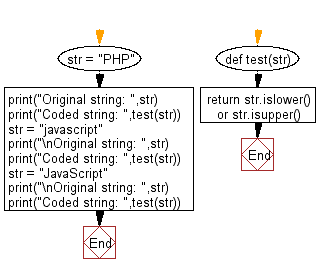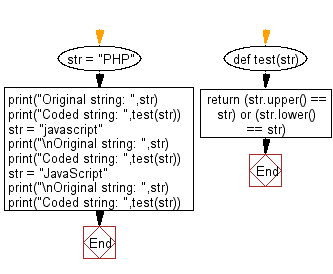﻿ Python: Check if a given string contains only lowercase or uppercase characters - w3resource# Python: Check if a given string contains only lowercase or uppercase characters

## Python Basic - 1: Exercise-122 with Solution

Write a Python program to check if a given string contains only lowercase or uppercase characters.

Sample Solution-1:

Python Code:

``````def test(str):
return str.islower() or str.isupper()

str = "PHP"
print("Original string: ",str)
print("Coded string: ",test(str))
str = "javascript"
print("\nOriginal string: ",str)
print("Coded string: ",test(str))
str = "JavaScript"
print("\nOriginal string: ",str)
print("Coded string: ",test(str))
``````

Sample Output:

```Original string:  PHP
Coded string:  True

Original string:  javascript
Coded string:  True

Original string:  JavaScript
Coded string:  False
```

Flowchart:Sample Solution-2:

Python Code:

``````def test(str):
return (str.upper() == str) or (str.lower() == str)

str = "PHP"
print("Original string: ",str)
print("Coded string: ",test(str))
str = "javascript"
print("\nOriginal string: ",str)
print("Coded string: ",test(str))
str = "JavaScript"
print("\nOriginal string: ",str)
print("Coded string: ",test(str))
``````

Sample Output:

```Original string:  PHP
Coded string:  True

Original string:  javascript
Coded string:  True

Original string:  JavaScript
Coded string:  False
```

Flowchart:Python Code Editor:

Have another way to solve this solution? Contribute your code (and comments) through Disqus.

What is the difficulty level of this exercise?

Test your Programming skills with w3resource's quiz.

﻿

## Python: Tips of the Day

What is the difference between Python's list methods append and extend?

append: Appends object at the end.

```x = [1, 2, 3]
x.append([4, 5])
print (x)
```

Output:

```[1, 2, 3, [4, 5]]
```

extend: Extends list by appending elements from the iterable.

```x = [1, 2, 3]
x.extend([4, 5])
print (x)
```

Output:

```[1, 2, 3, 4, 5]
```

Ref: https://bit.ly/2AZ6ZFq